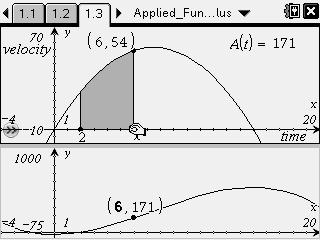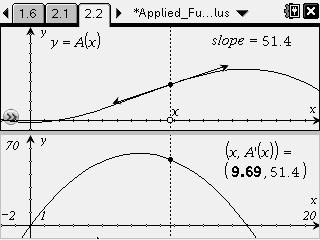# Activities

••• ##### Subject Area

• Math: Calculus: Fundamental Theorem

• ##### Author9-12

15 Minutes

• ##### Device
•TI-Nspire™ CX/CX II
•TI-Nspire™ CX CAS/CX II CAS
• TI-Nspire™ Navigator™
• TI-Nspire™
• TI-Nspire™ CAS
• ##### Software

TI-Nspire™ CAS

3.2

## Applied Fundamental Theorem of Calculus

#### Activity Overview

For a given function, students recognize the accumulation function as an antiderivative of the original function, and identify the graphical connections between a function and its accumulation function.

#### Key Steps

•Students will be able to identify the graphical connections between a function and its accumulation function for a road trip application problem. They will compare the graph of a velocity function to the graph of the accumulation function, A(t). Students will also use the graph to solve questions about the speed of a car at different times.

•Next, students will recognize the original function as the derivative of the accumulation function. Using information from the accumulation function, they will find the equation for the antiderivative of the velocity function. Students recognize this function, the accumulation, as the distance traveled on the road trip.